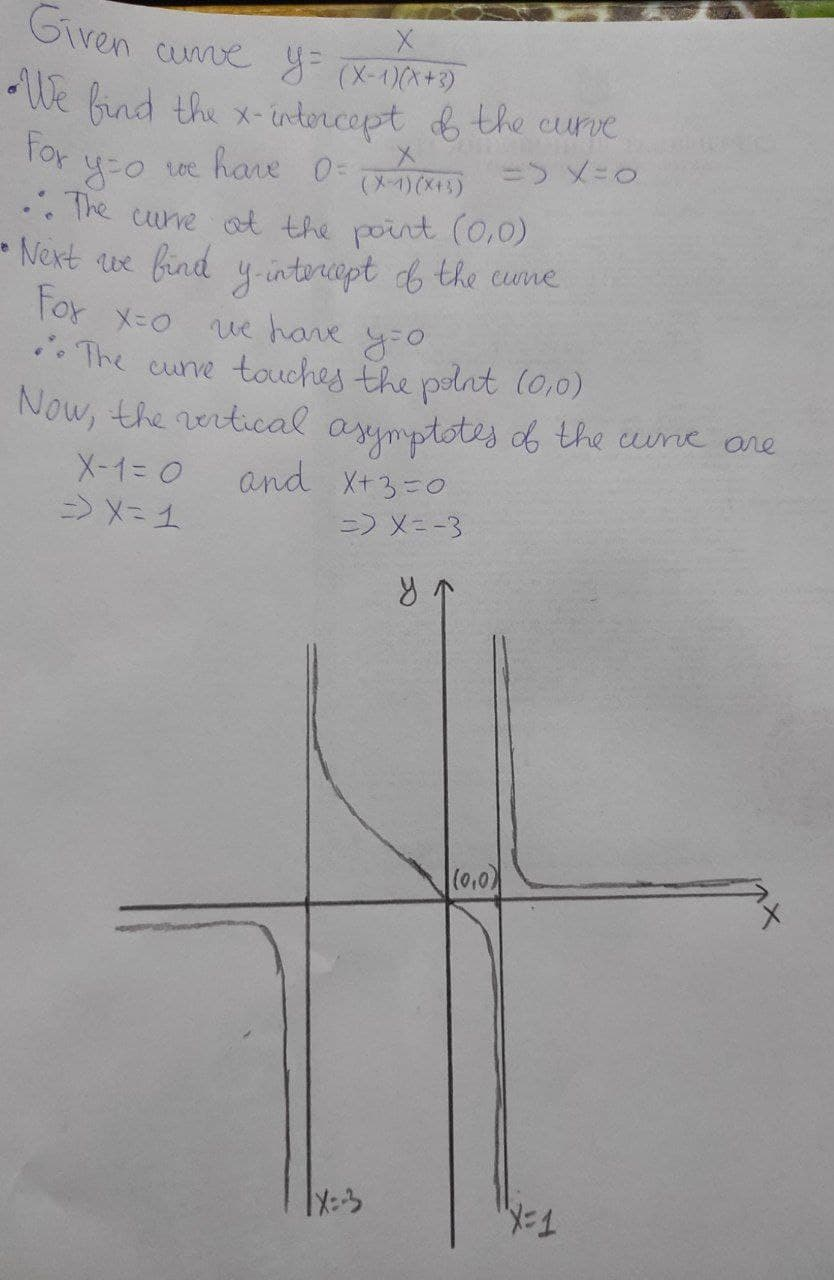# Trace the curve y = x / (x-1)(x+3)Maiclubk 2021-03-11 Answered
Trace the curve $$\displaystyle{y}=\frac{{x}}{{{x}-{1}}}{\left({x}+{3}\right)}$$

• Questions are typically answered in as fast as 30 minutes

### Plainmath recommends

• Get a detailed answer even on the hardest topics.
• Ask an expert for a step-by-step guidance to learn to do it yourself.lobeflepnoumni

We find the x-intercept of the curve
For $$y=o$$ we have $$\displaystyle={0}\frac{{x}}{{{\left({x}-{1}\right)}{\left({x}+{3}\right)}}}\Rightarrow{x}={0}$$
$$\displaystyle\therefore$$ The curve touches at the point (0,0)
ext we find y-intercept of the curve
For $$x=0$$ we have $$y=0$$
$$\displaystyle\therefore$$ The curve touches the point (0)
Now, the vertical asymptotes of the curve are
$$\displaystyle{x}-{1}={0}\Rightarrow{x}={1}$$
$$\displaystyle{x}+{3}={0}\Rightarrow{x}=-{3}$$